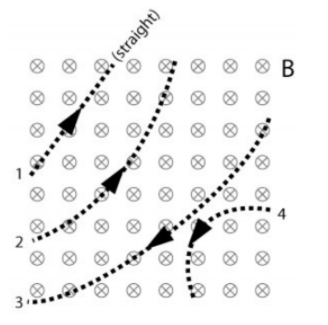# Problem: In the figure, a uniform magnetic field points into the page. (The magnetic field vectors are indicated by ⊗ symbols.). Four particles with the same mass follow the paths shown as they pass through the magnetic field with identical, constant speeds. What can we conclude about the charge on each particle? (Discuss sign, and relative magnitudes.)

###### FREE Expert Solution
95% (189 ratings)
###### Problem Details

In the figure, a uniform magnetic field points into the page. (The magnetic field vectors are indicated by ⊗ symbols.). Four particles with the same mass follow the paths shown as they pass through the magnetic field with identical, constant speeds. What can we conclude about the charge on each particle? (Discuss sign, and relative magnitudes.)